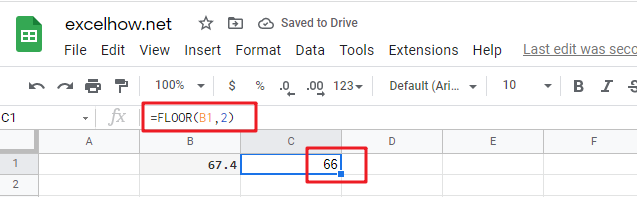This post will guide you how to use Google Sheets FLOOR function with syntax and examples.

## Description

The Google Sheets FLOOR function returns a number rounded down to the nearest multiple of significance. So it will return a rounded number.

The purpose of this function is to round a number down to the nearest specified multiple in google sheets. And its returned value is a rounded number.

The FLOOR function is a build-in function in Google Sheets and it is categorized as a MATH function.

## Syntax

The syntax of the FLOOR function is as below:

= FLOOR (number, significance)

Where the FLOOR function arguments are:

• Number -This is a required argument. The numeric value that you want to round.
• Significance – This is a required argument. The multiple to which you want to round

Note:

• If both above arguments is not numeric value, then the FLOOR function returns the #VALUE! Error value.
• If the number value is positive and the significance is negative, the FLOOR function returns the #NUM! Error value.
• If the significance argument is equal to 0, the FLOOR function returns the #DIV/0! Error.

## Google Sheets FLOOR Function Examples

The below examples will show you how to use google sheets FLOOR function to round a supplied number to the nearest multiple of a specified significance.

#1 to round the value in B1 Cell down to nearest multiple of 2, just using the following excel formula:

`=FLOOR(B1,2)`